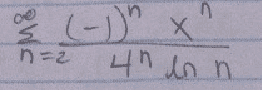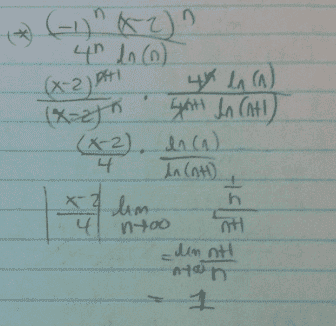# Finding the radius of convergence and interval of convergence

## Homework StatementThis is the question of mine that I'm having a little confusion about. I know the whole process in which you use the ratio test to determine the radius of convergence and using that you test the end points of the summation to see if they converge at the end points aswell.

However, I'm what I'm confused about is how to determine when to start the summation at 0 and what exactly does it mean when the summation starts at for example, 2.

I'm asking this because in the textbook that we are using for this course, sometimes they use the summation starting at 1 and sometimes starting at 0.

So how do we determine the the answer to the above question and if someone can just explain to me when to start the summation at a different number other than 0.

## Homework Equations

Ratio test eqn. lim n->infinity |An+1/An|

## The Attempt at a Solution

Took the limit and factored the absolute value of x however the summation starting at 2 is confusing me.

Mark44
Mentor
Since you have ln n in the denominator, n must be > 0 for its ln to be defined. Also, ln 1 = 0, so n must be > 1 so that you don't get division by zero. That's why the summation starts at n = 2. This changes nothing when you use the ratio test.

The first term in your series is
$$\frac{(-1)^2~x^2}{4^2 ln(2)}$$

Okay, thank you for that explination,
However, in the solutions they also put (x-2)^n instead of (x)^n
Any explination for that?

Mark44
Mentor
Okay, thank you for that explination,
However, in the solutions they also put (x-2)^n instead of (x)^n
Any explination for that?
None that I can think of. The power series you showed in the image is a Maclaurin series, a series in powers of x.

This is what I was refering to..Mark44
Mentor
Well, that's different from what you posted in the first image. This is a Taylor series in powers of (x - 2). For this series to converge, |x - 2| < 4.

Yeah okay now I understand, so now we apply the ratio test and find the interval of convergence and the radius of convergence, correct?

Mark44
Mentor
Yes.